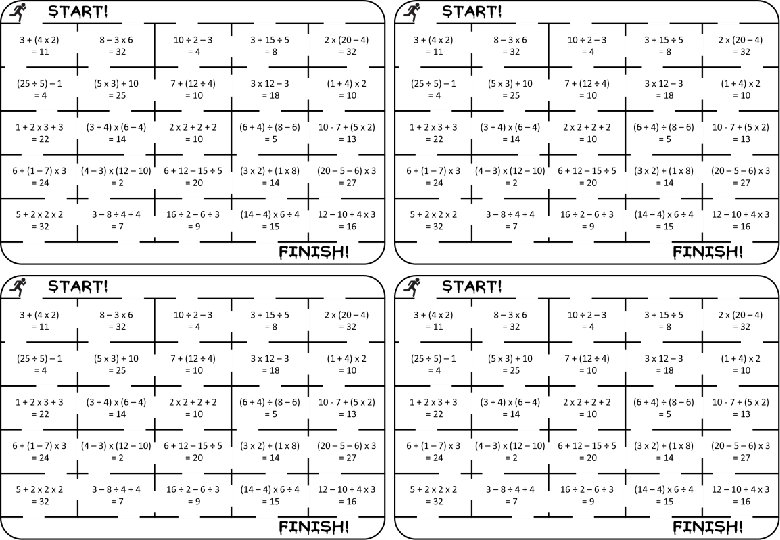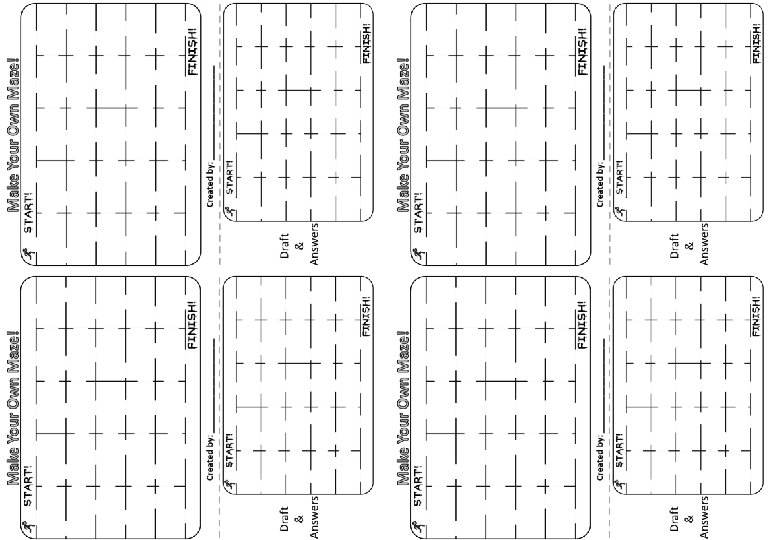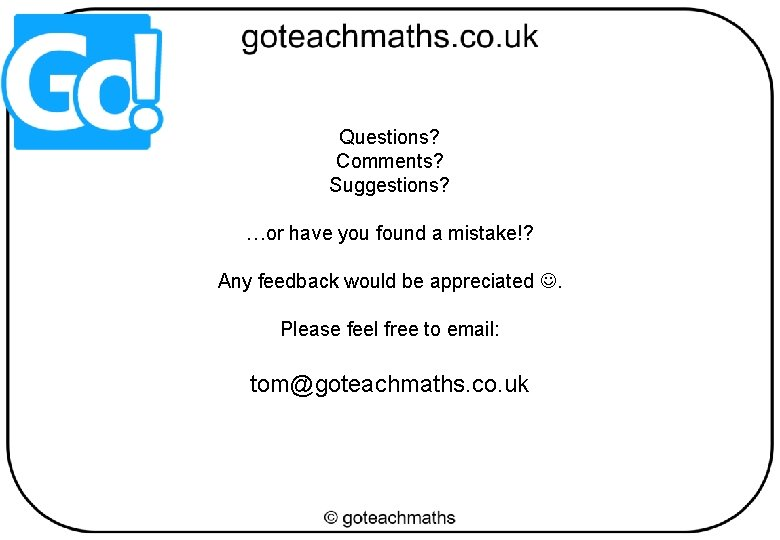# BIDMAS 4 True or False Maze Students need

• Slides: 11BIDMAS – 4 – True or False Maze Students need to find a route from the ‘Start’ to the ‘Finish’, only passing through rooms where the answer is correct. Students can only pass through gaps in the walls. Worksheets increase in difficulty and are in a variety of sizes/formats. A ‘Make Your Own’ for students is included.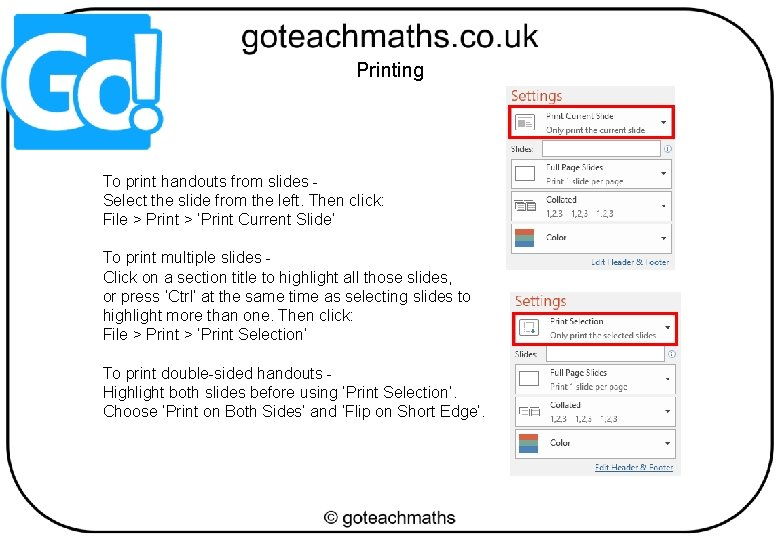Printing To print handouts from slides Select the slide from the left. Then click: File > Print > ‘Print Current Slide’ To print multiple slides Click on a section title to highlight all those slides, or press ‘Ctrl’ at the same time as selecting slides to highlight more than one. Then click: File > Print > ‘Print Selection’ To print double-sided handouts Highlight both slides before using ‘Print Selection’. Choose ‘Print on Both Sides’ and ‘Flip on Short Edge’.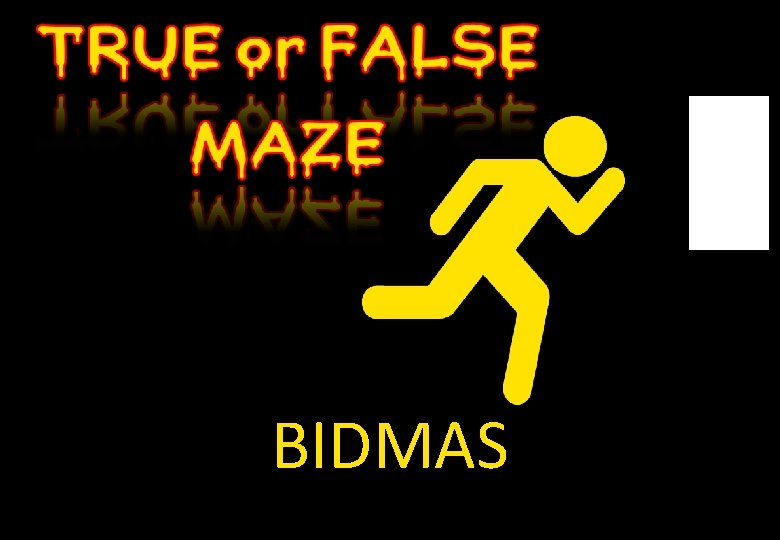BIDMAS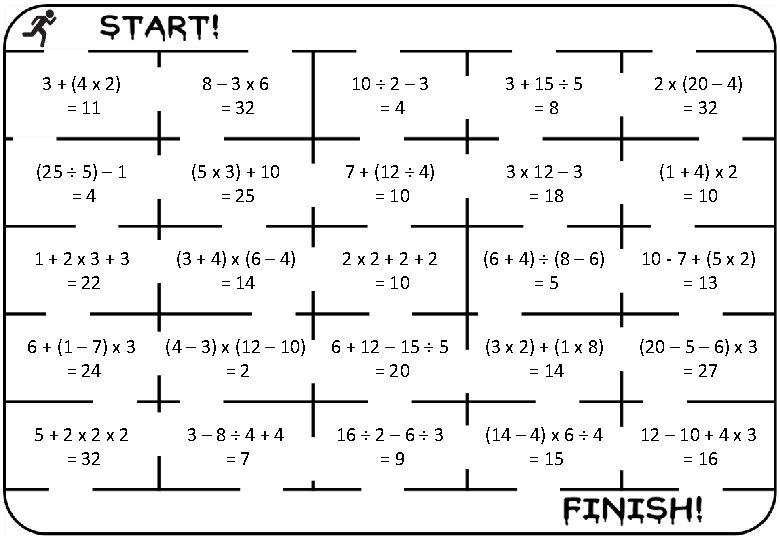3 + (4 x 2) = 11 8– 3 x 6 = 32 10 ÷ 2 – 3 =4 3 + 15 ÷ 5 =8 2 x (20 – 4) = 32 (25 ÷ 5) – 1 =4 (5 x 3) + 10 = 25 7 + (12 ÷ 4) = 10 3 x 12 – 3 = 18 (1 + 4) x 2 = 10 1+2 x 3+3 = 22 (3 + 4) x (6 – 4) = 14 2 x 2+2+2 = 10 (6 + 4) ÷ (8 – 6) =5 10 - 7 + (5 x 2) = 13 6 + (1 – 7) x 3 = 24 (4 – 3) x (12 – 10) =2 6 + 12 – 15 ÷ 5 = 20 (3 x 2) + (1 x 8) = 14 (20 – 5 – 6) x 3 = 27 5+2 x 2 x 2 = 32 3– 8÷ 4+4 =7 16 ÷ 2 – 6 ÷ 3 =9 (14 – 4) x 6 ÷ 4 = 15 12 – 10 + 4 x 3 = 16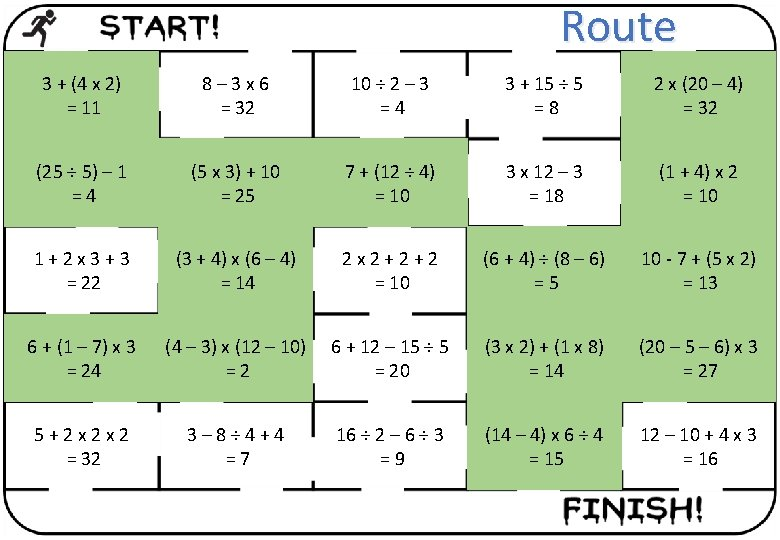Route 3 + (4 x 2) = 11 8– 3 x 6 = 32 10 ÷ 2 – 3 =4 3 + 15 ÷ 5 =8 2 x (20 – 4) = 32 (25 ÷ 5) – 1 =4 (5 x 3) + 10 = 25 7 + (12 ÷ 4) = 10 3 x 12 – 3 = 18 (1 + 4) x 2 = 10 1+2 x 3+3 = 22 (3 + 4) x (6 – 4) = 14 2 x 2+2+2 = 10 (6 + 4) ÷ (8 – 6) =5 10 - 7 + (5 x 2) = 13 6 + (1 – 7) x 3 = 24 (4 – 3) x (12 – 10) =2 6 + 12 – 15 ÷ 5 = 20 (3 x 2) + (1 x 8) = 14 (20 – 5 – 6) x 3 = 27 5+2 x 2 x 2 = 32 3– 8÷ 4+4 =7 16 ÷ 2 – 6 ÷ 3 =9 (14 – 4) x 6 ÷ 4 = 15 12 – 10 + 4 x 3 = 16